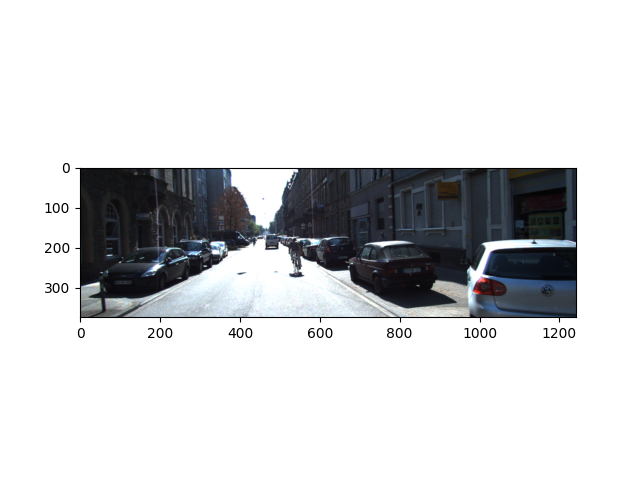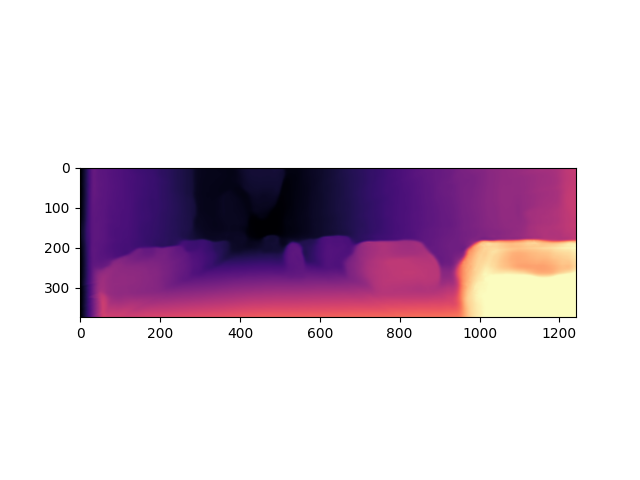# 01. Predict depth from a single image with pre-trained Monodepth2 models¶

This is a quick demo of using GluonCV Monodepth2 model for KITTI on real-world images. Please follow the installation guide to install MXNet and GluonCV if not yet.

```import numpy as np

import mxnet as mx
from mxnet.gluon.data.vision import transforms
import gluoncv
# using cpu
ctx = mx.cpu(0)
```

## Prepare the image¶

```url = 'https://raw.githubusercontent.com/KuangHaofei/GluonCV_Test/master/monodepthv2/tutorials/test_img.png'
filename = 'test_img.png'
```

Out:

```Downloading test_img.png from https://raw.githubusercontent.com/KuangHaofei/GluonCV_Test/master/monodepthv2/tutorials/test_img.png...

0%|          | 0/728 [00:00<?, ?KB/s]
729KB [00:00, 62213.06KB/s]
```

Then we load the image and visualize it,

```import PIL.Image as pil
img = pil.open(filename).convert('RGB')

from matplotlib import pyplot as plt
plt.imshow(img)
plt.show()
```We resize the image make it has the same input size with pretrained model, and transfer the image to NDArray,

```original_width, original_height = img.size
feed_height = 192
feed_width = 640

img = img.resize((feed_width, feed_height), pil.LANCZOS)
img = transforms.ToTensor()(mx.nd.array(img)).expand_dims(0).as_in_context(context=ctx)
```

## Load the pre-trained model and make prediction¶

Next, we get a pre-trained model from our model zoo,

```model = gluoncv.model_zoo.get_model('monodepth2_resnet18_kitti_stereo_640x192',
pretrained_base=False, ctx=ctx, pretrained=True)
```

Out:

```Downloading /root/.mxnet/models/monodepth2_resnet18_kitti_stereo_640x192-83eea4a9.zip from https://apache-mxnet.s3-accelerate.dualstack.amazonaws.com/gluon/models/monodepth2_resnet18_kitti_stereo_640x192-83eea4a9.zip...

0%|          | 0/70343 [00:00<?, ?KB/s]
0%|          | 101/70343 [00:00<01:28, 793.38KB/s]
1%|          | 518/70343 [00:00<00:30, 2257.21KB/s]
3%|3         | 2181/70343 [00:00<00:09, 7190.19KB/s]
11%|#1        | 7856/70343 [00:00<00:02, 23981.79KB/s]
20%|##        | 14291/70343 [00:00<00:01, 37146.42KB/s]
32%|###1      | 22159/70343 [00:00<00:00, 48307.49KB/s]
44%|####3     | 30615/70343 [00:00<00:00, 59307.70KB/s]
53%|#####3    | 37617/70343 [00:00<00:00, 62545.88KB/s]
65%|######5   | 46006/70343 [00:00<00:00, 68983.52KB/s]
76%|#######5  | 53136/70343 [00:01<00:00, 69680.23KB/s]
88%|########7 | 61615/70343 [00:01<00:00, 72331.49KB/s]
99%|#########8| 69592/70343 [00:01<00:00, 48262.71KB/s]
70344KB [00:01, 46948.06KB/s]
```

We directly make disparity map predictions on the image, and resize it to input size

```outputs = model.predict(img)
disp = outputs[("disp", 0)]
disp_resized = mx.nd.contrib.BilinearResize2D(disp, height=original_height, width=original_width)
```

In the end, we add normalized color map for visualizing the predicted disparity map,

```import matplotlib as mpl
import matplotlib.cm as cm
disp_resized_np = disp_resized.squeeze().as_in_context(mx.cpu()).asnumpy()
vmax = np.percentile(disp_resized_np, 95)
normalizer = mpl.colors.Normalize(vmin=disp_resized_np.min(), vmax=vmax)
mapper = cm.ScalarMappable(norm=normalizer, cmap='magma')
colormapped_im = (mapper.to_rgba(disp_resized_np)[:, :, :3] * 255).astype(np.uint8)
im = pil.fromarray(colormapped_im)
im.save('test_output.png')

import matplotlib.image as mpimg